## How to create dynamic top 10 or n list in Excel?

A top list is used to rank the companies or individuals based on values. Supposing, you have a list of students’ grades of a class, now, you want to create a dynamic top 10 list of the students as below screenshot shown. In this article, I will introduce some formulas for creating a top 10 or n list in an Excel worksheet.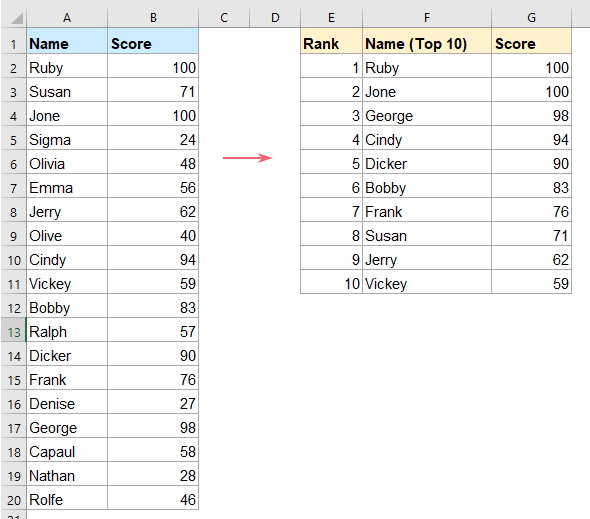#### Create a dynamic top 10 list in Excel

In Excel 2019 and earlier versions, to extract the top 10 list or top 10 list with criteria, you should apply the following formulas:

##### Formulas to create a dynamic top 10 list

1. First, you should extract the top 10 values from the data range, please use the below formula into a blank cell - G2, and drag the fill handle down to get the top 10 values, see screenshot:

=LARGE(\$B\$2:\$B\$20,ROWS(B\$2:B2))
Note: In this formula, B2:B20 is the data list where you want to get the top 10 values, and B2 is the first cell of the data list.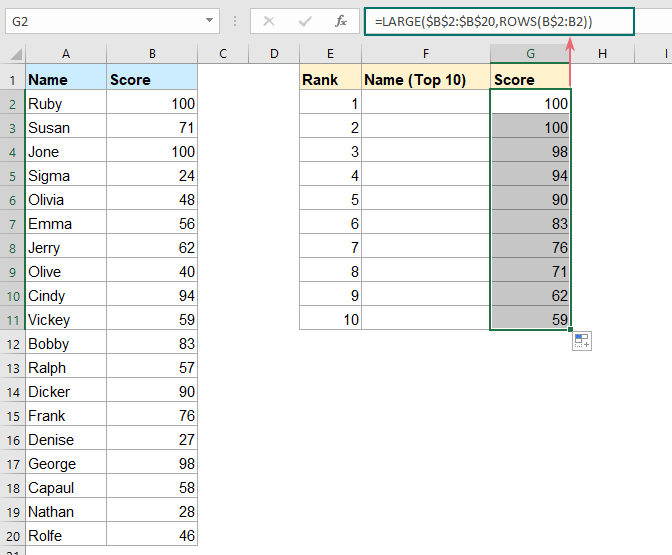2. Then, go on applying the following formula into cell - F2, and press Ctrl + Shift + Enter keys together to get the first result, see screenshot:

=INDEX(\$A\$2:\$A\$20,SMALL(IF(\$B\$2:\$B\$20=G2,ROW(\$B\$2:\$B\$20)-ROW(\$B\$1)),COUNTIF(\$G\$2:G2,G2)))
Note: In the above formula, A2:A20 is the data list that you want to pull the names of top 10 values from, B2:B20 is the data list that contains all the values, G2 is the cell of the largest value extracted from the column B and B1 is the header cell of the value list.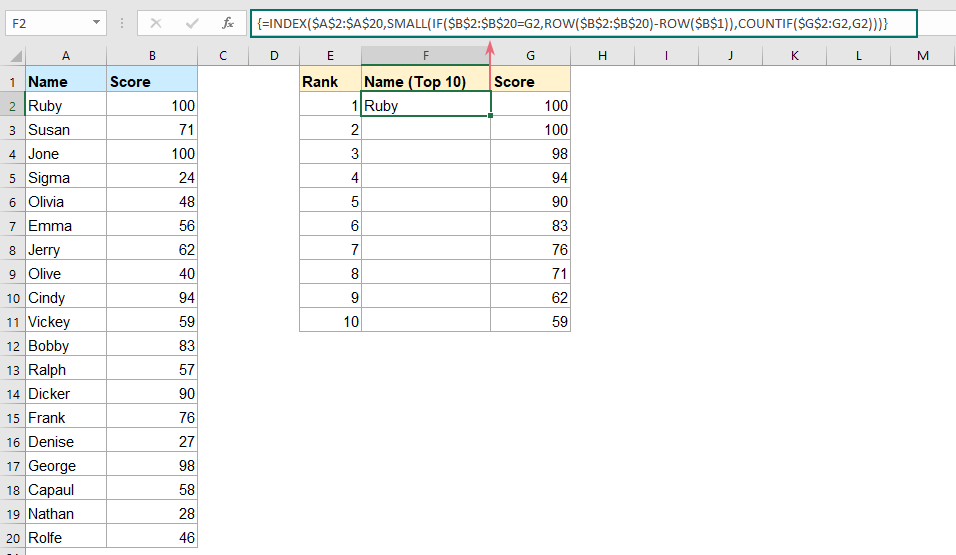3. After getting the first result, select the formula cell and drag the fill handle down to get other names at once, see screenshot: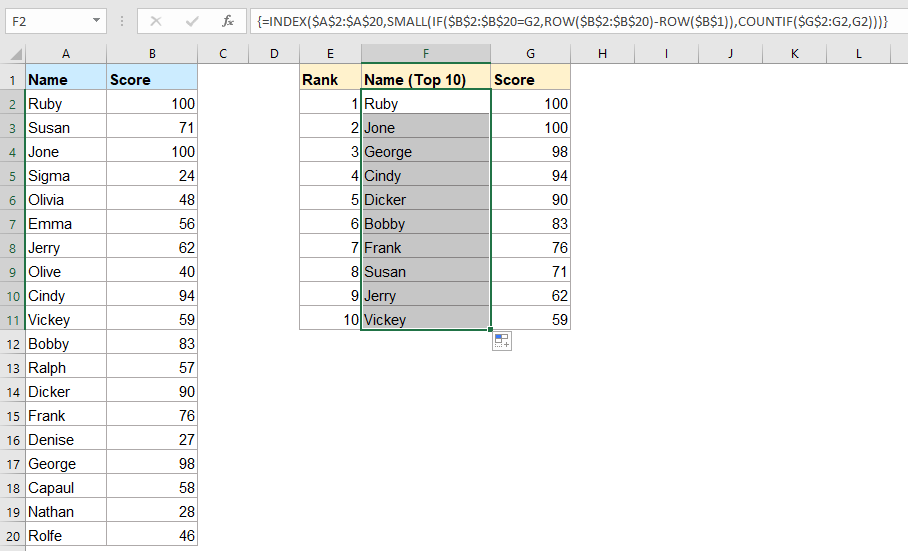##### Formulas to create a dynamic top 10 list with criteria

Sometimes, you may need to get the top 10 list based on criteria. For example, to get the top 10 names and scores of Class1 as below screenshot shown.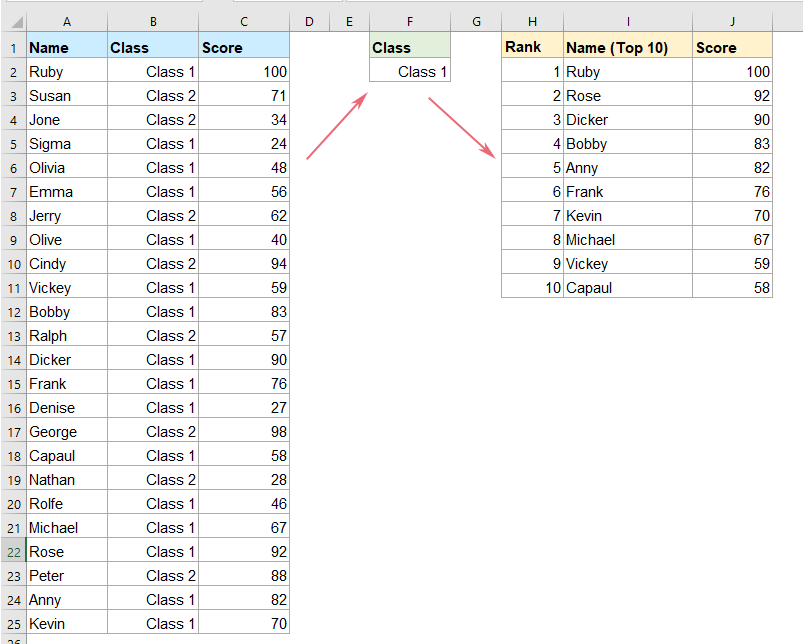1. To get the top 10 list, you should also need to extract the top 10 scores with this formula:

=LARGE(IF(\$B\$2:\$B\$25=\$F\$2,\$C\$2:\$C\$25),ROW(I2)-ROW(I\$1))

2. And then press Ctrl + Shift + Enter keys together to get the first result, then drag this formula down to display other values, see screenshot: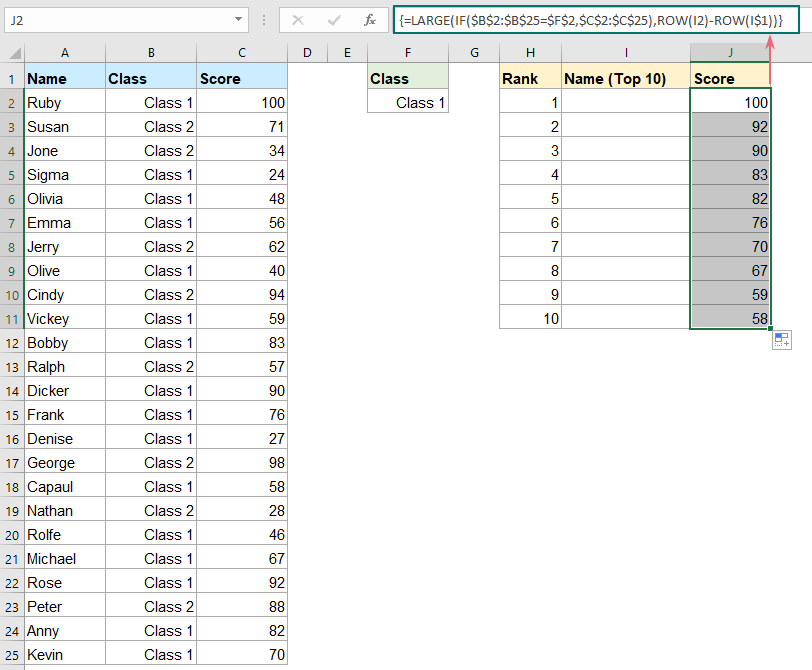3. Then, please copy and paste the following formula into cell - I2, and press Ctrl + Shift + Enter keys simultaneously to extract the first result, and then drag this formula to fill it to other cells, and the top 10 names are displayed as below screenshot shown:

=INDEX(\$A\$2:\$A\$25,SMALL(IF((\$C\$2:\$C\$25=J2)*(\$B\$2:\$B\$25=\$F\$2),ROW(\$C\$2:\$C\$25)-ROW(\$C\$1)),COUNTIF(J2:\$J\$2,J2)))

#### Create a dynamic top 10 list in Office 365

The above formulas may be difficult for us to understand, if you are using Office 365, with its INDEX, SORT and SEQUENCE functions, you can create simple formulas for completing this task.

##### Formula to create a dynamic top 10 list

To get the10 list of data, please use the below formula:

=INDEX(SORT(A2:B20,2,-1),SEQUENCE(10),{1,2})

And then, just press Enter key, all the data of the top 10 list are displayed at once, see screenshot:Tips:

SORT function:

=SORT(array, [sort_index], [sort_order], [by_col])

• array: The range of cells that you want to sort;
• [sort_index]: The column or row number to sort to the array by. For example, to sort by the second column of the data range, the sort index would be 2;
• [sort_order]: The number 1 (or omitted) indicates to sort in ascending order; the number -1, sort in descending order;
• [by_col]: The direction of sorting. TRUE, sort by columns, FALSE or omitted, sort by rows.

In the formula, we use the SORT function as this:

SORT(A2:B20,2,-1): Means to sort the cell range A2:A20 on the second column in descending order.

SEQUENCE function:

=SEQUENCE(rows, [columns], [start], [step])

• rows: The number of rows to return,
• [columns]: The number of columns to return. If omitted, it will return a single column.
• [start]: The first number in the sequence. If omitted, it will start at 1.
• [step]: The increment between each number. If excluded, each increment will be 1.

In this formula, we use SEQUENCE(10) to create a list from 1 to 10.

At last, put the both SORT and SEQUENCE functions into the INDEX function: =INDEX(SORT(A2:B20,2,-1),SEQUENCE(10),{1,2}), this will return the first 10 records from the data range and return columns 1 and 2.

##### Formula to create a dynamic top 10 list with criteria

To display the top 10 list with criteria, you should embed the FILTER function into the SORT function as this:

=INDEX(SORT(FILTER(A2:C25,B2:B25=F2),3,-1),SEQUENCE(10),{1,3})

And just press Enter key, all the data of the top 10 list based on the given criteria are displayed at once, see screenshot: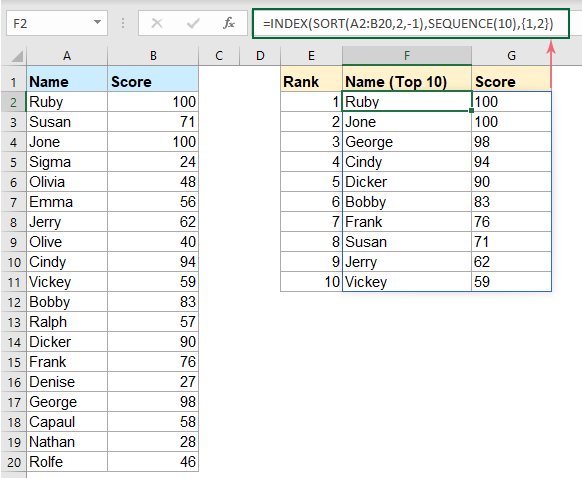Tips:

FILTER function:

=FILTER(array, include, [if_empty])

• array: The range of cells to filter.
• include: The condition you use to filter the arrayto get an array of TRUE or FALSE result, so that the TRUE values will be retained in the filter.
• [if_empty]: The value to be displayed if no matching results are returned.

In this formula: =FILTER(A2:C25,B2:B25=F2) is used to filter in the range A2:C25, where the values from B2:B25 equal to the specific cell F2.

### Best Office Productivity Tools

 Popular Features: Find, Highlight or Identify Duplicates   |  Delete Blank Rows   |  Combine Columns or Cells without Losing Data   |   Round without Formula ... Super Lookup: Multiple Criteria VLookup  |   Multiple Value VLookup  |   VLookup Across Multiple Sheets   |   Fuzzy Lookup .... Advanced Drop-down List: Quickly Create Drop Down List   |  Dependent Drop Down List   |  Multi-select Drop Down List .... Column Manager: Add a Specific Number of Columns  |  Move Columns  |  Toggle Visibility Status of Hidden Columns  |  Compare Ranges & Columns ... Featured Features: Grid Focus   |  Design View   |   Big Formula Bar   |  Workbook & Sheet Manager   |  Resource Library (Auto Text)   |  Date Picker   |  Combine Worksheets   |  Encrypt/Decrypt Cells   |  Send Emails by List   |  Super Filter   |   Special Filter (filter bold/italic/strikethrough...) ... Top 15 Toolsets:  12 Text Tools (Add Text, Remove Characters, ...)   |   50+ Chart Types (Gantt Chart, ...)   |   40+ Practical Formulas (Calculate age based on birthday, ...)   |   19 Insertion Tools (Insert QR Code, Insert Picture from Path, ...)   |   12 Conversion Tools (Numbers to Words, Currency Conversion, ...)   |   7 Merge & Split Tools (Advanced Combine Rows, Split Cells, ...)   |   ... and more

Supercharge Your Excel Skills with Kutools for Excel, and Experience Efficiency Like Never Before. Kutools for Excel Offers Over 300 Advanced Features to Boost Productivity and Save Time.  Click Here to Get The Feature You Need The Most...#### Office Tab Brings Tabbed interface to Office, and Make Your Work Much Easier

• Enable tabbed editing and reading in Word, Excel, PowerPoint, Publisher, Access, Visio and Project.
• Open and create multiple documents in new tabs of the same window, rather than in new windows.
• Increases your productivity by 50%, and reduces hundreds of mouse clicks for you every day!